How Cheenta works to ensure student success?
Explore the Back-Story

# ISI Entrance 2007 - B.Math Subjective Paper| Problems & SolutionsHere, you will find all the questions of ISI Entrance Paper 2007 from Indian Statistical Institute's B. Math Entrance. You will also get the solutions soon of all the previous year problems.

Problem 1:

Let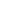be a positive integer . Ifhas odd number of divisors ( other thanand) , then show thatis a perfect square .

Problem 2:

Let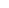and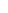be two non-zero rational numbers such that the equation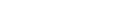has a non-zero solution in rational numbers . Prove that for any rational number, there is a solution of the equation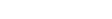.

Problem 3:

For a natural number n>1 , consider the n-1 points on the unit circle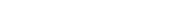. Show that the product of the distances of these points fromis.

Problem 4:

Let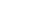be an isosceles triangle with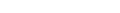. Let P be a point inside the trianglesuch that the sum of the distances of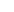to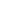andis. Describe the locus of all such points inside triangle.

Problem 5:

Let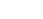be a polynomial with integer coefficients of degree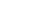.(a) Ifand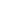are two integers such that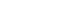and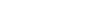, then prove that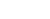divides 2.(b) Prove that the number of distinct integer roots of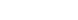is at most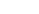.

Problem 6:

In ISI club each member is on two committees and any two committees have exactly one member in common . There are 5 committees . How many members does ISI club have?

Problem 7:

Let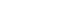. Prove that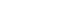.
Solution

Problem 8:

Let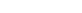be a continuous function such that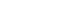has no real solution. Prove that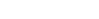has no real solution.
Solution

Problem 9:

In a group of five people any two are either friends or enemies , no three of them are friends of each other and no three of them are enemies of each other . Prove that every person in this group has exactly two friends .

Problem 10:

The eleven members of a cricket team are numbered 1,2,...,11. In how many ways can the entire cricket team sit on the eleven chairs arranged around a circular table so that the numbers of any two adjacent players differ by one or two ?

Here, you will find all the questions of ISI Entrance Paper 2007 from Indian Statistical Institute's B. Math Entrance. You will also get the solutions soon of all the previous year problems.

Problem 1:

Letbe a positive integer . Ifhas odd number of divisors ( other thanand) , then show thatis a perfect square .

Problem 2:

Letandbe two non-zero rational numbers such that the equationhas a non-zero solution in rational numbers . Prove that for any rational number, there is a solution of the equation.

Problem 3:

For a natural number n>1 , consider the n-1 points on the unit circle. Show that the product of the distances of these points fromis.

Problem 4:

Letbe an isosceles triangle with. Let P be a point inside the trianglesuch that the sum of the distances oftoandis. Describe the locus of all such points inside triangle.

Problem 5:

Letbe a polynomial with integer coefficients of degree.(a) Ifandare two integers such thatand, then prove thatdivides 2.(b) Prove that the number of distinct integer roots ofis at most.

Problem 6:

In ISI club each member is on two committees and any two committees have exactly one member in common . There are 5 committees . How many members does ISI club have?

Problem 7:

Let. Prove that.
Solution

Problem 8:

Letbe a continuous function such thathas no real solution. Prove thathas no real solution.
Solution

Problem 9:

In a group of five people any two are either friends or enemies , no three of them are friends of each other and no three of them are enemies of each other . Prove that every person in this group has exactly two friends .

Problem 10:

The eleven members of a cricket team are numbered 1,2,...,11. In how many ways can the entire cricket team sit on the eleven chairs arranged around a circular table so that the numbers of any two adjacent players differ by one or two ?

This site uses Akismet to reduce spam. Learn how your comment data is processed.

### 3 comments on “ISI Entrance 2007 - B.Math Subjective Paper| Problems & Solutions”

1.Rajarshi Chatterjee says:

please send me the solution of the forth no question.

2.esha says:

3.esha says:

### Knowledge Partner